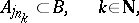# Nikodým convergence theorem

A theorem [a6], [a7], [a4] saying that for a pointwise convergent sequence $\{ \mu _ { n } \}$ of countably additive measures (cf. Measure) defined on a $\sigma$-algebra $\Sigma$, i.e., $\operatorname { lim } _ { n \rightarrow \infty } \mu _ { n } ( E ) = \mu ( E )$, $E \in \Sigma$:

i) the limit $m$ is a countably additive measure;

ii) $\{ \mu _ { n } \}$ is uniformly $\sigma$-additive. As is well-known, the Nikodým convergence theorem for measures fails in general for algebras of sets. But there are convergence theorems in which the initial convergence conditions are imposed on certain subfamilies of a given $\sigma$-algebra; those subfamilies need not be $\sigma$-algebras. The following definitions are useful [a2], [a9], [a8]:

SCP) An algebra $\mathcal{A}$ has the sequential completeness property if each disjoint sequence $\{ E _ { n } \}$ from $\mathcal{A}$ has a subsequence $\{ E _ { n_j} \}$ whose union is in $\mathcal{A}$.

SIP) An algebra $\mathcal{A}$ has the subsequentional interpolation property if for each subsequence $\{ A _ { j n } \}$ of each disjoint sequence $\{ A _ { j } \}$ from $\mathcal{A}$ there are a subsequence $\{ A _ { j n _ { k } } \}$ and a set $B \in \mathcal{A}$ such thatand $A _ { j } \cap B = \emptyset$ for $j \in \mathbf{N} \backslash \{ j _ { n_k } : k \in \mathbf{N} \}$.

The Nikodým convergence theorem holds on algebras with SCP) and SIP).

A famous result of J. Dieudonné [a3], Prop. 8, and A. Grothendieck [a5], p. 150, states that for compact metric spaces, respectively locally compact spaces, convergence of a sequence of regular Borel measures on every open set implies convergence on all Borel sets (cf. also Borel set).

Many related results can be found in [a1], [a8], where the method of diagonal theorems is used instead of the commonly used Baire category theorem (see [a4], [a10] and Diagonal theorem).

How to Cite This Entry:
Nikodým convergence theorem. Encyclopedia of Mathematics. URL: http://encyclopediaofmath.org/index.php?title=Nikod%C3%BDm_convergence_theorem&oldid=50739
This article was adapted from an original article by E. Pap (originator), which appeared in Encyclopedia of Mathematics - ISBN 1402006098. See original article